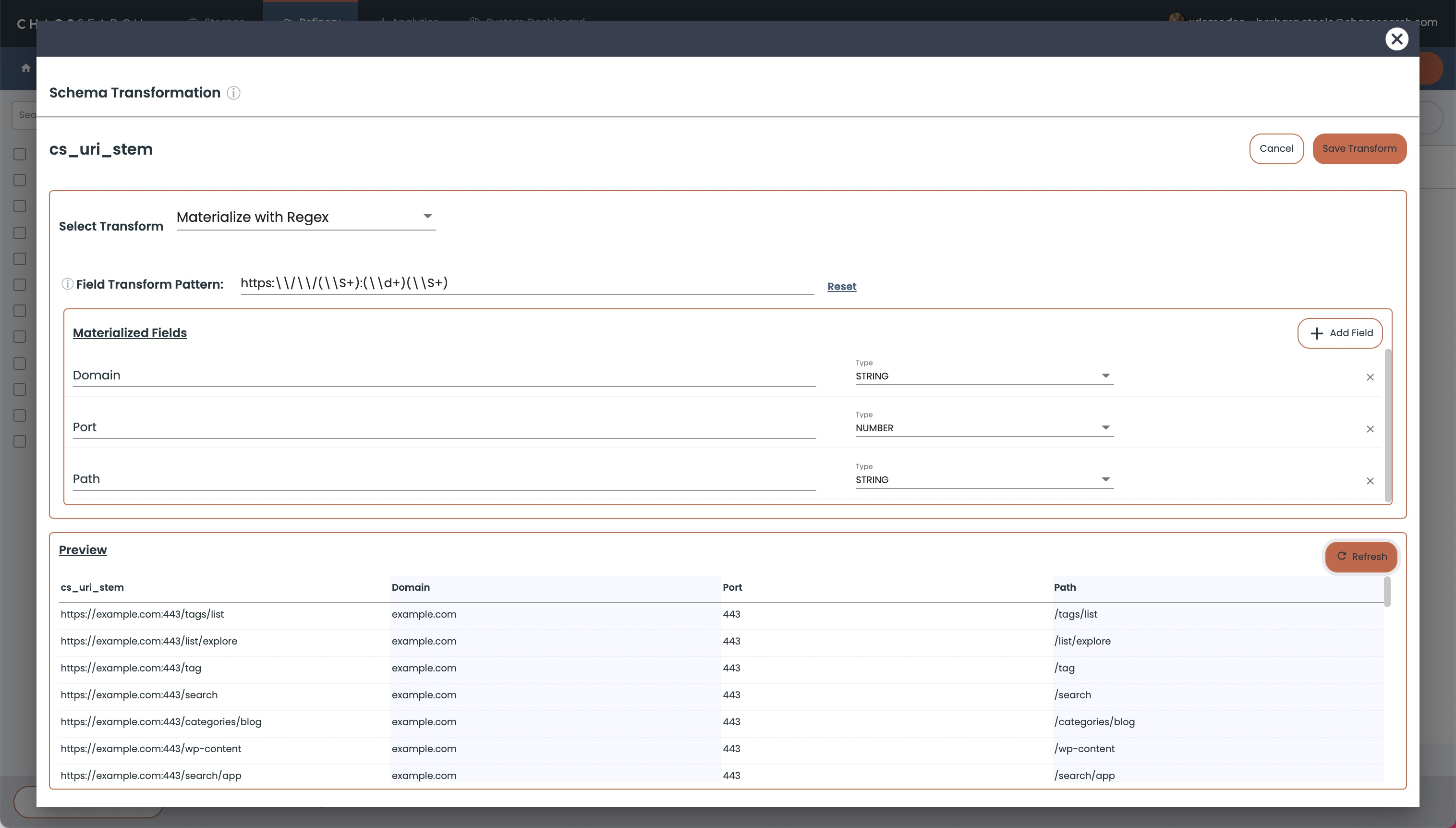# Refinery Transformation Regex Reference

Review these sample regex patterns for the Chaos Refinery®.

Some sample regular expression (regex) patterns for common transformations. If you have some popular patterns for transformations, we welcome your suggestions for additions to this reference.

# Refinery Regex Examples:

## AWS ELB Logs – Parse Body Field

``````(?:(\\w+:\\w+:\\w+:\\w+-\\w+-\\d+:\\d{12}+)):(\\S+)\\s(?:(\\D\\w+\\D)(\\S+)") (\\S+) (\\S+) (\\S+) (\\S+) (\\S+) (\\S+) (\\S+) (\\S+) (\\S+) (\\S+) (\\S+)
``````

Common field names added, 15 fields in total:

body.arn
targetGroup
body.root
body.traceID
body.domain_name
body.chosen_cert_arn
body.matched_rule_priority
body.request_creation_time
body.actions_executed
body.redirect_url
body.error_reason
body.target_port_list
body.target_status_code_list
body.classification
body.classification_reason

## IP Address Transformation

The following pattern transforms an IP address into its component fields of address class, subnet, network, and host.

``````(\\d+)\\.(\\d+)\\.(\\d+)\\.(\\d+)
``````

A sample screen shows this transformation: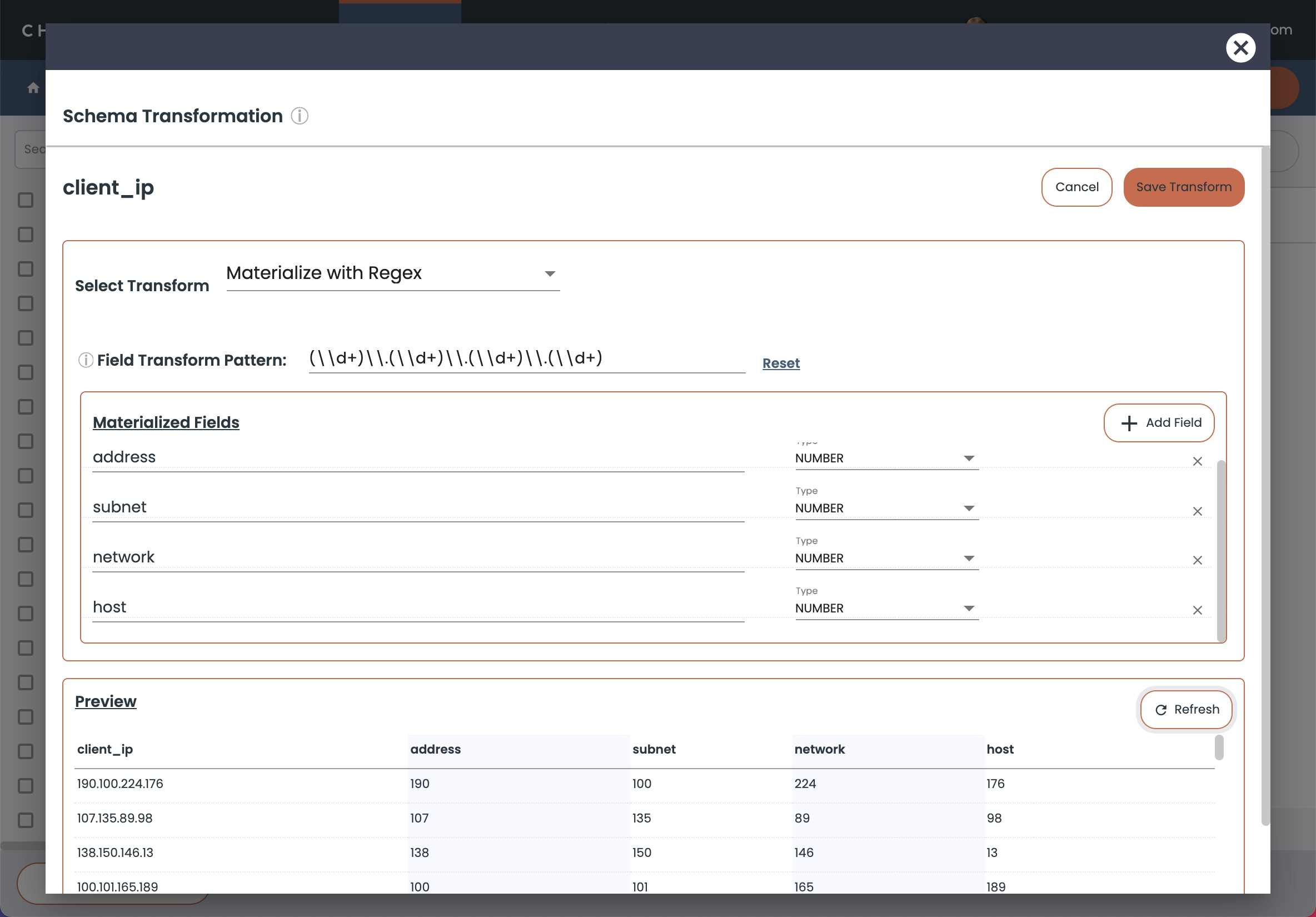## IP Address and Port Separation

The following pattern could be used to transform an 1.2.3.4:1234 address form column into two fields, one that contains the IP address and one for the port:

``````(^(?:[0-9]{1,3}\\.){3}[0-9]{1,3}):(\\d+)
``````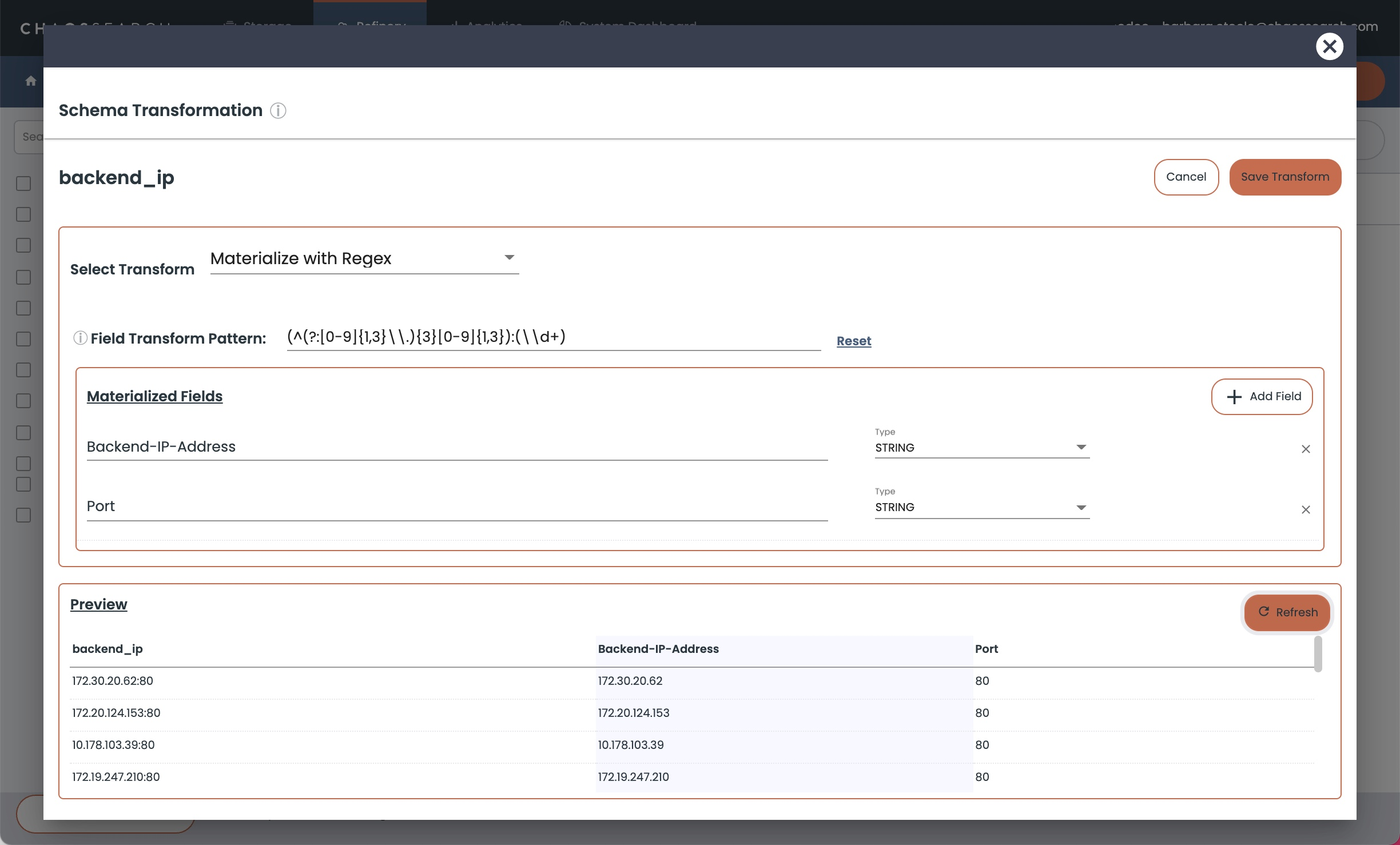## Request Breakdown

``````(\\w+) (\\S+) (\\w+.\\d.\\d)
``````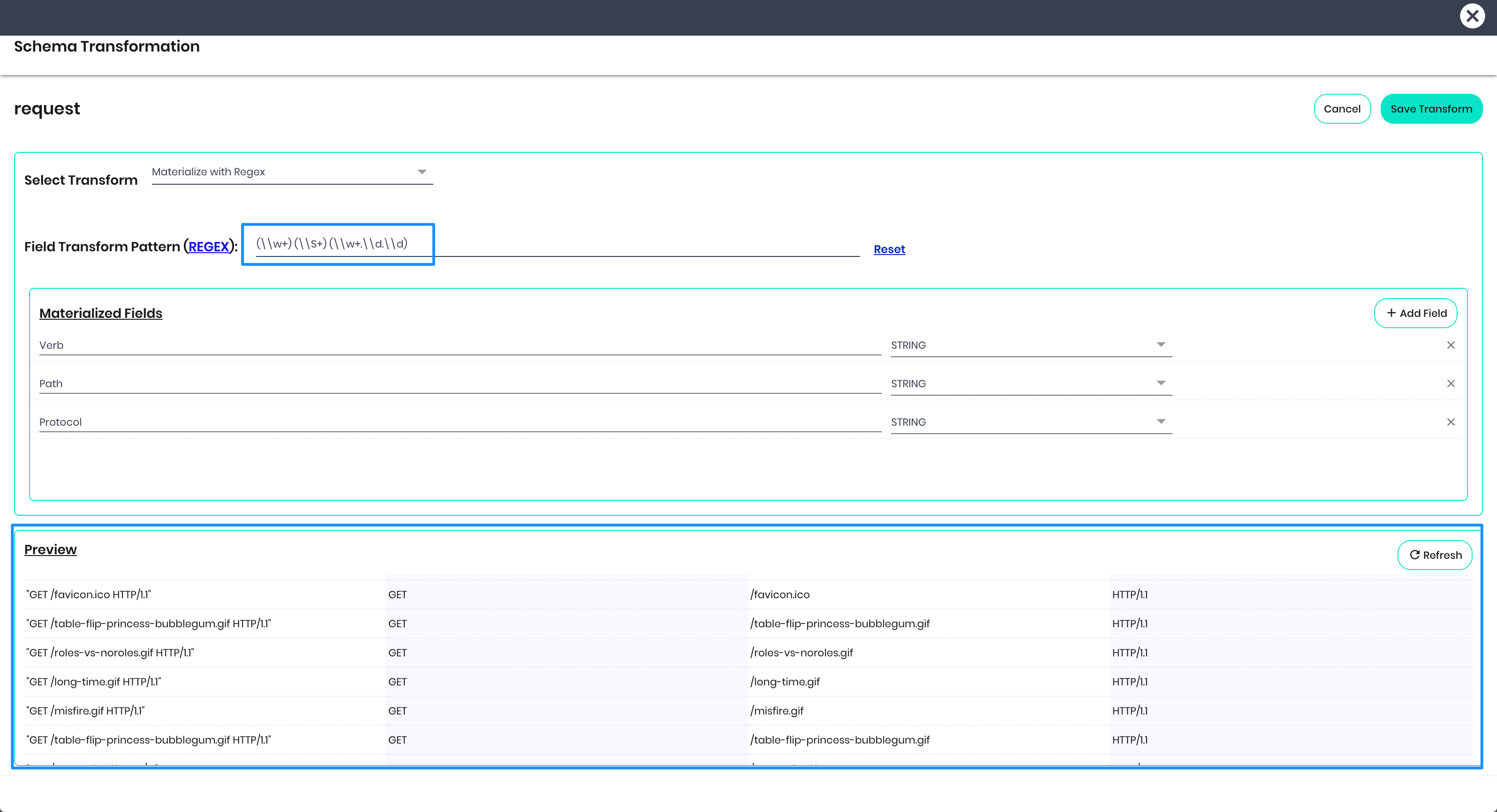## AWS ARN KMS Separation

``````(\\w+):(\\w+):(\\w+):(\\w+-\\w+-\\d+):(\\d{12}+):(\\S+)
``````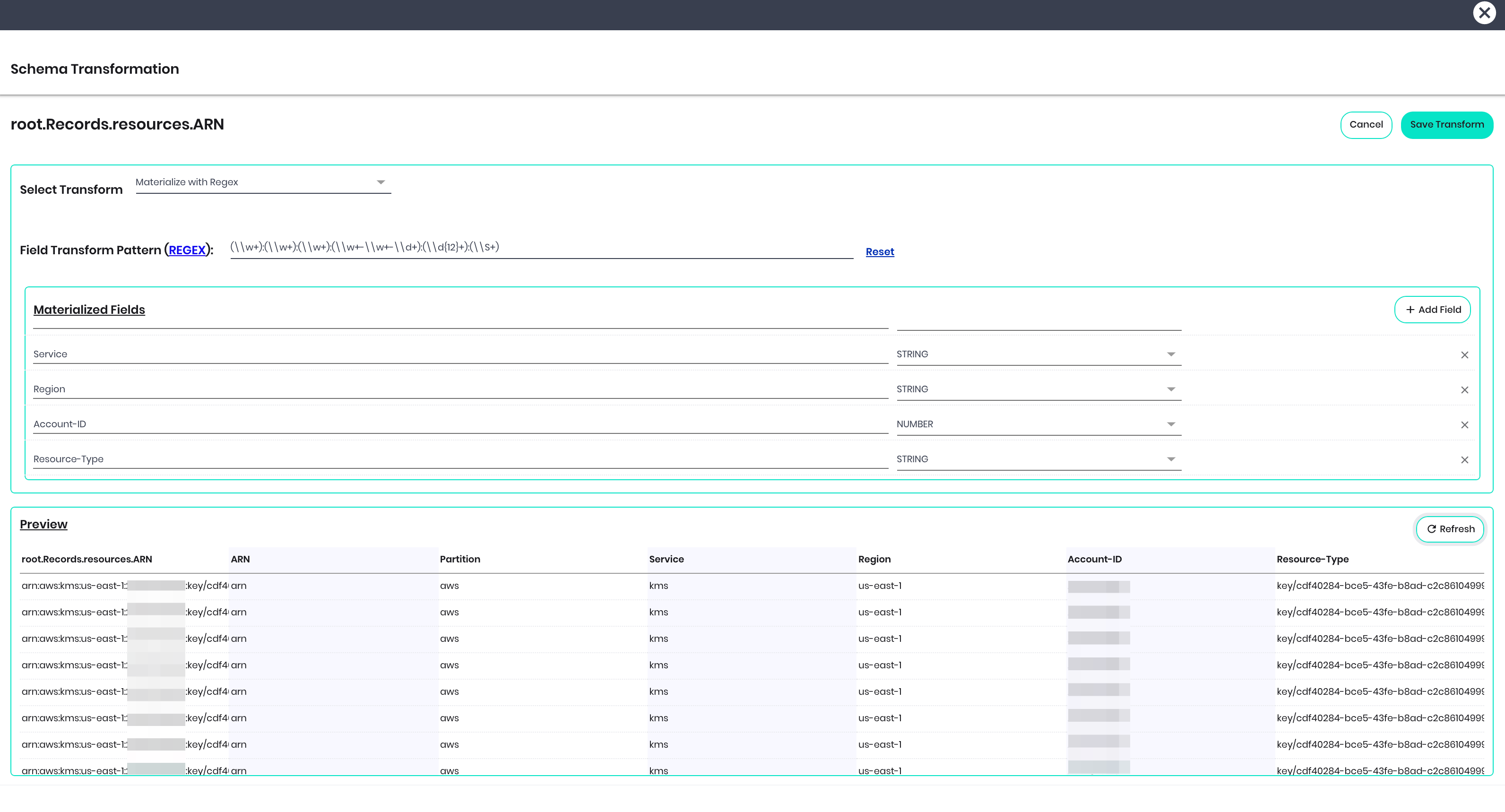## URI Stem

``````https:\\/\\/(\\S+):(\\d+)(\\S+)
``````